# Beta kernel and transformed kernel

This Thursday I will give a talk at Laval University, on “Beta kernel and transformed kernel : applications to copula density estimation and quantile estimation“. This time, I will talk at the department of Mathematics and Statistics (13:30 at the pavillon Adrien-Pouliot). “Because copulas have bounded support (the unit square in dimension 2), standard kernel based estimators of densities are (multiplicatively) biased on borders and in corners of the support. Two techniques can be used to avoid that underestimation: Beta kernels and Transformed kernel. We will describe and discuss those two techniques in the first part of the talk. Then, we will see that it is possible to combine those two techniques to get nice estimator of several quantities (e.g. quantiles): transform the data to get on the unit interval – using a transformed kernel – then estimate the (transformed) quantile on [0,1] using a beta kernel, then get back on the initial support. As we will see on simulations, that technique can be better than standard quantile estimators, especially when data are heavy tailed.” Slides can be downloaded here.• kernel based density estimation

Kernel based estimation are a popular (and natural) technique to estimate densities.  It is simply and extension of the moving histogram:so we count how many observations are a the neighborhood of the point where we want to estimate the density of the distribution. Then it is natural so consider a smoothing function, i.e. instead of a step function (either observations are close enough, or not), it is possible to give weights to observations, which will be a decreasing function of the distance,With a smooth kernel, we have a smooth estimation of the densityThen it is possible to play on the bandwidth, either to get a more accurate estimation of the density, but not that smooth (small bias but large variance),or a smoother one (large bias, but small variance),In R, it is simply

> X=rnorm(100)
> (D=density(X))

Call:
density.default(x = X)

Data: X (100 obs.);	Bandwidth 'bw' = 0.3548

x                   y
Min.   :-3.910799   Min.   :0.0001265
1st Qu.:-1.959098   1st Qu.:0.0108900
Median :-0.007397   Median :0.0513358
Mean   :-0.007397   Mean   :0.1279645
3rd Qu.: 1.944303   3rd Qu.:0.2641952
Max.   : 3.896004   Max.   :0.3828215

> plot(D$x,D$y)
• Beta kernel

The idea of Beta kernel is to consider kernels having support [0,1]. In the univariate case,whereis the density of a Beta distribution, i.e.For additional material, I have uploaded some R code to fit copula densities using beta kernels,

library(copula)
beta.kernel.copula.surface = function (u,v,bx,by,p) {
s = seq(1/p, len=(p-1), by=1/p)
mat = matrix(0,nrow = p-1, ncol = p-1)
for (i in 1:(p-1)) {
a = s[i]
for (j in 1:(p-1)) {
b = s[j]
mat[i,j] = sum(dbeta(a,u/bx,(1-u)/bx) *
dbeta(b,v/by,(1-v)/by)) / length(u)
} }
return(data.matrix(mat)) }

Then we can used it to see what we get on a simulated sample

library(copula)
COPULA = frankCopula(param=5, dim = 2)
X = rcopula(n=1000,COPULA)
p0 = 26
Z= beta.kernel.copula.surface(X[,1],X[,2],bx=.01,by=.01,p=p0)
u = seq(1/p0, len=(p0-1), by=1/p0)
box=FALSE,zlim=c(0,6))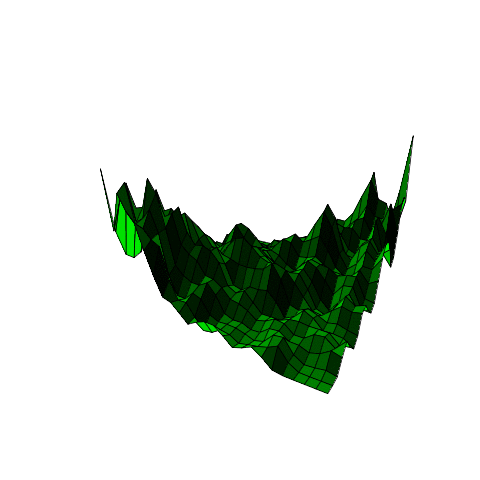(yes, the surface is changing… to illustrate the impact of the bandwidth on the estimation).

• transformed kernel estimationI the talk, I will also mention the transformed Kernel estimate, as introduced in the book on L1 density estimation by Luc Devroye and Laszlo Györfi (the book can be downloaded here). I probably spend a few minutes on the original chapter, in order to provide another application of that techniques (not only to estimate copula densities, but here to estimate quantiles of heavy tailed distribution). In the univariate case, the R code is the following (here I consider two transformation, the quantile function of the Gaussian distribution, and the quantile function of the Student distribution with 3 degrees of freedom),

set.seed(1)
sample=rbeta(100,4,3)

transfN = function(x){
Y=qnorm(sample)
f=density(Y,from=-4,to=4,n=2001)
ny=sum(f$x<=qnorm(x)); g=f$y[ny]/dnorm(qnorm(x))
return(g)
}

df0=3

transfT = function(x){
Y=qt(sample,df=df0)
f=density(Y,from=-4,to=4,n=2001)
ny=sum(f$x<=qt(x,3)); g=f$y[ny]/dt(qt(x,df=df0),df=df0)
return(g)
}

tN=Vectorize(transfN)
tT=Vectorize(transfT)

u=seq(.01,.99,by=.01)
vN=tN(u)
vT=tT(u)
plot(u,vN,type="l",lwd=3,col="blue")
lines(u,vT,lwd=3,col="green")
lines(u,dbeta(u,4,3),col="red",lty=2)

The density estimation is the following,(the red dotted line is the true density, since we work on a simulated sample). Now, let us get back on the initial chapter,In the book, this is introduced as follows,The original idea we add it to use this kernel based estimator for copulas, i.e. since we can estimate densities in high dimension with unbounded support, usingthe idea is to transform marginal observations,and to use the fact that the associated copula density can be writtento derive an intuitive estimator for the copula densityAn important issue is how do we choose the transformationAnd Luc Devroye and Laszlo Györfi mention that this can be used to deal with extremes.well, extremes are introduced through bumps (which is not the way I would have been dealing with extremes)and note that several results can be derived on those bumps,e.g.Then, there is an interesting discussion about estimating the optimal transformationand I will prove that this can be an extremely interesting idea, for instance to estimate quantiles of heavy tailed distribution, if we use also the beta kernel estimator on the unit interval. This idea was developed in a paper with Abder Oulidi, online here.

Remark: actually, in the book, an additional reference is mentioned,but I have never been able to find a copy… if anyone has one, I’d be glad to read it…

# Some stylized facts about large risk covers

A couple of weeks ago, David Cummins (here) was giving a talk in Laval University. And we’ve seen a series of extremely interesting graphs and figures about catastrophe reinsurance market, as well as Cat Bonds prices. The first one was the rate one line index for catastrophe reinsurance (the rate on line is the excess of loss premium expressed as a percentage of the reinsurance cover), from Guy Carpenter (2010, page 10 here).Following hurricane Andrew in 1992, prices went up quite high. But following hurricane Katrina (which is, so far, the most costly insured disaster following the second World War, with a cost exceeding 70 billions US$– 2008$ – while Andrew was only 24 billions – again 2008 $), the bump is much smaller. I though cycles where much larger in the reinsurance industry. Then there was a discussion about cat bond pricing, with a graph from Lane Financial (2010, page 13, here) with the ratio premium over expected lossThis is extremely interesting, even if it is only about cat bond, and not about reinsurance covers. Usually, when we introduce premium principles in actuarial courses, we start with the pure premium, i.e.Then we explain that with such a price, ruin probability is certain (with an infinite time horizon), so we need to add a safety margin, and a standard idea (but that can be criticized since the expected value has – usually – nothing to do with the variability) is to add a loading proportional to the pure premium. Then the premium isFor small risks, like motor insurance, the loading is not huge. Actually, if risks have finite variance, it can be obtained simply using the central limit theorem (but I’ll get back on that point in a couple of weeks). Here, we see that loadingcan be large (up to 400% in 2009). An finally an updated graph with a comparison between BB corporate bondscoupon, and BB catastrophe bonds coupon,(I guess the source is again Morton Lane). I found surprising the recent gap (following Katrina) between the two spreads. I guess financial market started to be scared and understood that catastrophes are not that rare…. I wonder what 2008 and 2009 prices looked like. # Comparer des taux sur deux populations Lors du dernier cours, nous avions évoqué les tests de comparaison des moyennes et des proportions sur deux échantillons. Pour cela, nous avions vu qu’il était possible d’utiliser la statistiqueLa statistique doit alors suivre, sous l’hypothèse où les proportions sont égales une loi normale centrée réduite. Sous R, c’est assez facile à implémenter. Afin d’illustrer, utiliser l’information suivanteBon, en l’état on n’a pas vraiment un taux, mais un nombre de tués sur les routes. On peut introduire la probabilité d’avoir un tué sur les routes par minute. Le code sous R serait alors > prop.test(x=c(308,308/1.027), n=c(31*24*60,31*24*60), alt="two.sided") 2-sample test for equality of proportions with continuity correction data: c(308, 308/1.027) out of c(31 * 24 * 60, 31 * 24 * 60) X-squared = 0.0834, df = 1, p-value = 0.7727 alternative hypothesis: two.sided 95 percent confidence interval: -0.0009198487 0.0012826342 sample estimates: prop 1 prop 2 0.006899642 0.006718249 Autrement dit, la probabilité d’avoir un accident, à la minute, est sensiblement le même, avec une p-value de 77%. On notera sur le graphique suivant que l’impact du pas de temps a globalement peu d’importance….)L’idéal serait d’avoir des données détaillées, mais il faudra attendre un peu pour ça… # Time horizon in forecasting, and rules of thumbI recently received an email about forecasting and rules of thumb. “Dans la profession […] se transmet une règle empirique qui voudrait que l’on prenne un historique du double de l’horizon de prévision : 20 ans de données pour une prévision à 10 ans, etc… Je souhaite savoir si cette règle n’aurait pas, par hasard, un fondement théorique quitte à ce que le rapport ne soit pas de 2 pour 1, mais de 3 pour 1 ou de 1 pour 1 par exemple.” To summarize briefly, the rule is to consider a 2-1 ratio for the period of observation vs. forecast horizon. And the interesting question is if there are justifications for such a rule… At first, I remembered a rules of thumb, from the book by Box and Jenkins, which states that it is meaningless to look at autocorrelations when lags exceed the sample size over 6. So with 12 years of data, autocorrelations with a lag higher than two years are useless. But it is not what is mentioned here. So I looked at some dataset, and some standard time series models. • It depends on the series It might obvious… but if it is the case, it means that it will be difficult to have a general rule of thumb. Consider e.g. the number of airline passengers, library(forecast) X = AirPassengers ETS = ets(X) plot(forecast(ETS,h=length(X)/2)) or some sales in a big store,or car casualties in France, or the temperature in Nottingham Castle,or the water level at Lack Hurron, or the flow of the Nile river,or see also here for forecasting techniques in demography. Actually, in the case of life insurance, actuaries have to forecast future demography, i.e. try to assess death rates of those who currently purchase retirement contracts, who might be 20 years old. So they have to forecast death rate until 2100, say. One the one hand, it sounds difficult to make forecast over a century (it is already difficult for climate, I guess it is even more complex for human life). On the other hand, a 2-1 ratio means that we have to use data from 1800… Here again, it is difficult to justify that mortality in the 1850 could be interesting to say anything about mortality in 2050. So I guess it will be difficult to justify the use of general rules of thumb…. • It depends on the model Consider the following (simulated) series. Several models can be fitted. And the shape on the forecast (and the forecast error) will depend on the model considered. The benchmark can be the model without any dynamics, i.e. we assume that observations are i.i.d. Or more classically, assume that it is simple a white noise, i.e. an i.i.d centered process. Then the forecast is the following,With that kind of assumption, we see that the 2-1 ratio is useless since we can get forecasts up to any horizon…. But that does not seem very robust. For instance, if we consider exponential smoothing techniques, we can obtainWhich is rather different. And with the 2-1 ratio, obviously, there is a lot of uncertainty at the end ! It would be even worst if we assume that we look at a random walk. Because actually a dozen models – at least – can be considered, from ARIMA, seasonal ARIMA, Holt Winters, Exponential Smoothing, etc…So I do not see any theoretical justification of that rule of thumb. Obviously, the maximum horizon can not be extremely far away if the series is non-stationary, with a very irregular pattern, and with a lot of noise… So we’re back at the beginning. If anyone is willing to share his or her experience, comments are open. # Talk at Laval University at the Actuarial Seminar I was last Friday at Laval University for a conference by David Cummins and Mary Weiss (here). I will be back tomorrow, this time to give a talk, on “distorting probabilities in actuarial science” (the talk will be extremely close to the one I gave at McGill in November). “In this talk, we will first get back on properties of distortion operators for pricing financial and insurance risks. Based on the dual version of the expected utility framework, we will see how distorted risk measures have been introduced, from VaR and TVaR, to Esscher premium and Wang’s measures. Then we will discuss extensions in higher dimension. We will discuss tail properties of distorted copulas (in the particular case of Archimedean copulas). A natural application will be aging problems (in survival analysis or in credit risk).” Slides can be downloaded from here. This talk can be seen as a first part, the second one behing the talk I will give in 15 days, again at Laval University, but this time for the Seminar of Statistics. The talk will be on “Beta kernel and transformed kernel : applications to quantile estimation, and copula density estimation“.# Variable annuities is not a systemic risk ?The Geneva Association just published on its website an interesting report on variable annuities and systemic risk (online here). Based on a definition of potentially systemically risky activities, on interconnectedness or substitutability, the report claims that since “none of the criteria is triggered”, variables annuities is “not a potentially systemically risk activity”. Even if “short-term effects are conceivable”. I guess it is a diplomatic way to say it… Note that a series of slides can also be downloaded (there) on insurance and systemic risk. But that deserves a more detailed post. # STT2700, estimation, tests et coupes du mondeMercredi, pour le dernier cours, nous allons revenir sur l’estimation, les tests, et plus généralement sur la modélisation statistique. Pour cela, j’avais pensé travailler sur les nombres de buts marqués, par match, lors de différentes coupes du monde de soccer (1982, 1998 et 2010). Je ne mets pas l’intégralité du code aujourd’hui, l’idée est pour l’instant de mettre en ligne des données qui serviront à répondre aux questions qui seront posées mercredi. Le code (accompagné – éventuellement – d’explications théoriques) sera posté par la suite. soccer1982=read.table("http://freakonometrics.free.fr/soccer1982") S82=(soccer1982$V1+soccer1982$V2) soccer1998=read.table("http://freakonometrics.free.fr/soccer1998") S98=(soccer1998$V1+soccer1998$V2) soccer2010=read.table("http://freakonometrics.free.fr/soccer2010") S10=(soccer2010$V1+soccer2010\$V3)

Les boxplot associés à ces trois échantillons sont les suivants,On va se poser des questions autour de ces données, par exemple voir s’il est vraisemblance (ou pas) que le nombre moyen de but dans un match (avant prolongation, s’il y en a eu). On peut commencé par essayer de se demander quel modèle utiliser. Classiquement, la loi de Poisson est la plus utilisée (en plus, c’est la seule loi qui est autorisée lorsqu’on publie un billet le 1er avril). Les histogrammes sont les suivants

boxplot(S82,S98,S10,col=c("red","yellow","blue"),
label=("1982","1998","2010"))
hist(S82,breaks=0:11,col="red")
hist(S98,breaks=0:11,col="yellow")
hist(S10,breaks=0:11,col="blue")Si on compare les fonctions de répartition empiriques à celles de lois de Poisson ajustées par maximum de vraisemblance, on obtient, pour la coupe du monde de 1982et pour celle de 2010,Visuellement, l’ajustement semble relativement bon, surtout en 2010. On peut aussi faire un test du chi-deux,

> library(vcd)
> (GF=goodfit(S10,type="poisson"))

Observed and fitted values for poisson distribution
with parameters estimated by ML

count observed     fitted
0        7  6.6409703
1       17 15.0459484
2       13 17.0442384
3       14 12.8719508
4        7  7.2907534
5        5  3.3036226
6        0  1.2474617
7        1  0.4037543

> summary(GF)

Goodness-of-fit test for poisson distribution

X^2 df  P(> X^2)
Likelihood Ratio 5.586765  5 0.3485255

On voit que l’on accepte l’ajustement par une loi de Poisson. Pour ceux qui veulent une visualisation, sur la figure ci-dessous, on a la densité d’une loi du chi-deux. Le premier trait vertical est la valeur observée, et l’aire jaune est alors la p-value (qui excède largement 5%). En rouge on a 5%, donc le second trait vertical est la borne de la région critique associé au test pour une erreur de première espèce valant 5%,On peut ensuite faire plein de tests.  On suppose que . On va pouvoir tester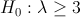contre une hypothèse alternative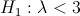Comme on a une hypothèse sur la loi des observations qui semble robuste, on peut utiliser un test de type rapport de vraisemblance.
On peut aussi faire un test de la forme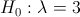contre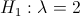(histoire de tester des hypothèses simples – qui ont une interprétation). Sinon, comme ce qui nous intéresse, c’est de savoir si on a plus de trois buts par matchs, on peut définir la variable binomiale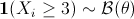, en notant que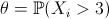est une proportion – donc facile à tester – qui nous intéresse ici compte tenu du problème que l’on cherchera à résoudre. On pourra alors tester, par exemple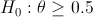contre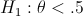Ces derniers tests sont alors facile à mettre en œuvre,

> Z=(S10>=3)*1
> prop.test(sum(Z),length(Z),p=1/2,alternative="less")

1-sample proportions test with continuity correction

data:  sum(Z) out of length(Z), null probability 1/2
X-squared = 1.2656, df = 1, p-value = 0.1303
alternative hypothesis: true p is less than 0.5
95 percent confidence interval:
0.0000000 0.5322764
sample estimates:
p
0.421875

On peut aussi faire des tests de moyenne sur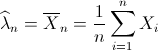Un test de l’hypothèsecontre une hypothèse alternatives’écrit alors
> t.test(S10,mu=3,alternative ="less")

One Sample t-test

data:  S10
t = -3.7763, df = 63, p-value = 0.0001775
alternative hypothesis: true mean is less than 3
95 percent confidence interval:
-Inf 2.590273
sample estimates:
mean of x
2.265625
Mais on l’aura l’occasion de revoir tous les points du cours, y compris aller peut être un peu plus loin, par exemple sur la comparaison de moyenne entre échantillons,
> t.test(S82,S98,var.equal=FALSE)

Welch Two Sample t-test

data:  S82 and S98
t = 0.427, df = 85.266, p-value = 0.6704
alternative hypothesis: true difference in means is not equal to 0
95 percent confidence interval:
-0.5503669  0.8514658
sample estimates:
mean of x mean of y
2.807692  2.657143

# Fin des débats sur la valeur de πLa rumeur avait agité pas mal de monde en août dernier lors du congrès international de mathématiques, à Hyderabad (même si à l’époque, ce sont surtout les médailles Fields qui avaient retenu toute l’attention, en France en tous les cas), mais finalement le communiqué de l’Union Internationale de Mathématiques (IMU) est finalement tombé hier soir: à compter du 1er juillet, π sera officiellement égal à 4.
Pour ceux qui ont suivi les débats (je n’ai eu que des bruits de couloir), Microsoft avait augmenté la pression ces derniers mois, quand le cap des 5000 milliards de décimales avait été franchi (ici). Comme l’avait dit Bill Gates avant le congrès à Hyderabad, bientôt la moitié de ma mémoire d’un processeur sera dédiée à stocker les décimales de π.Et comme il l’avait souligné “la recherche sur les décimales de π allant plus vite que la recherche des améliorations de Windows, nous allons rapidement faire face à un choc informatique sans précédant”. Il avait comparé la situation au bug de l’an 2000 (en ajoutant que – comme il y a 11 ans – la transition se fera, selon lui, en douceur).
Dans le communiqué de l’IMU, il est mentionné que “π gardera son interprétation originelle « περίμετρος »” (périmètre en grec), et en particulier, l’IMU utilise la définition géométrique suivante:Mais en quoi cela peut avoir un intérêt pour mon blog (hormis faire mon frimeur pour montrer que j’ai compris une démonstration géométrique). Tout simplement car π joue un rôle central en statistique et en probabilité (même si on a souvent tendance à l’oublier) au travers de la loi normale ! Pareil en risk management, y compris pour va valorisation des options, mais aussi le capital réglementaire calculé dans les accords de Bâle. Les banquiers se sont réjouit de l’annonce de l’IMU hier soir, car pour ceux qui l’auraient oublié, la loi normale intervient partout en finance . En particulier dans les calculs de quantiles. Rappelons que la densité s’écrit(pour les utilisateurs de R, la version 2.12.3. sera lancée exceptionnellement plus tôt afin d’intégrer cette mise à jour – et je crois que MSExcel a prévu un addins qui sera bientôt en ligne, comme pour le passage à l’an 2000).
Si on regarde “à la main” ce que vont devenir les probabilités de dépassement de seuils, on obtient

> 1-pnorm(2)
 0.02275013
> integrate(f=function(x){exp(-x^2/2)/sqrt(2*pi)},2,+Inf)
0.02275013 with absolute error < 1.5e-05
> integrate(f=function(x){exp(-x^2/2)/sqrt(2*4)},2,+Inf)
0.02016178 with absolute error < 1.3e-05

autrement dit, la probabilité de dépasser 2 va passer de 2.27% à 2.02%. Pour les quantiles à 99.5% (utilisées par les institutions financières), on a

> qnorm4(.995)
 2.543701
> qnorm(.995)
 2.575829Autrement dit la baisse est finalement relativement faible. On peut alors s’attendre à une (très) légère baisse des fonds propres des banques et institutions financières (même si pour l’instant, les instances de Bâle ne se sont pas encore prononcées).